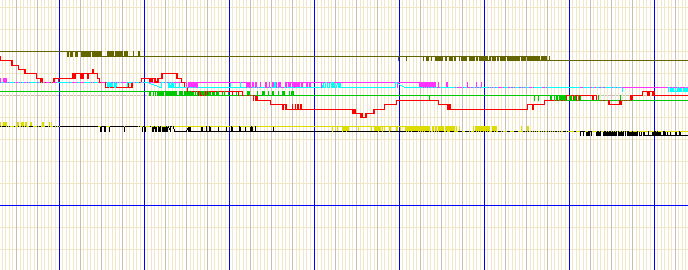# Oversampling + Timer triggered ADC

I wrote this code a little while back and have found it quite useful for sampling at defined sampling frequencies. It over samples to get 12 bit values from the 10 bit ADC. The basics of oversampling is that for every n bits you want to add you have to sample at 2^(2*n) times the desired sampling frequency. So, if you want to get 12-bit data from a 10 bit adc at 10Hz you would have to sample 2^4 = 16 times faster (160Hz). This is exactly what this code does for you. To initialize you must first declare the analog pin as an input as you would do normally, then call the setupADC() function. If you wanted to sample channel 2 at 40Hz (actually sampling at 640Hz) you would declare A2 as an input and then call: setupADC(2,40). Then you can do what ever you please with the data by changing the code after the comment "DEAL WITH DATA HERE" in the adc interrupt service routine. The code below is setup to simply output the data over a serial port connection every time the oversample buffer fills. This is all interrupt driven so you main loop is open to do whatever in between sampling events.

``````/*

#define BUFFER_SIZE 16 // For 12 Bit ADC data

volatile uint32_t result[BUFFER_SIZE];
volatile int i = 0;
volatile uint32_t sum=0;

Argument 1: uint8_t channel must be between 0 and 7
Argument 2: int frequency must be integer from 1 to 600
WARNING! Any value above 600 is likely to result in a loss
of data and could result in a reduced accuracy of ADC
conversions
*/
{
cli();
TCCR1A = _BV(COM1B1);
TCCR1B = _BV(CS11)| _BV(CS10) | _BV(WGM12);
uint32_t clock = 250000;
uint16_t counts = clock/(BUFFER_SIZE*frequency);
OCR1B = counts;

TIMSK1 = _BV(OCIE1B);
sei();
}

{
i=++i&(BUFFER_SIZE-1);
for(int j=0;j<BUFFER_SIZE;j++)
{
sum+=result[j];
}
if(i==0)
{
/****DEAL WITH DATA HERE*****/
sum = sum>>2;
Serial.println(sum,DEC);
}
sum=0;
TCNT1=0;
}
ISR(TIMER1_COMPB_vect)
{
}
``````

It's not as simple as that - over sampling can give better time-discrimination or voltage discrimination or both, and its critical how much random noise is present - in the absence of noise over-sampling only gives more time information. Too much noise and it makes the accuracy worse. Right amount of noise and the averaging both reveals the missing fractional sample data and cancels out the noise.
A saw-tooth wave of amplitude 0.5LSB works even better than random noise.

Pic is of some battery-voltage readings from Arduino-compatibles - here the noise present is significantly less than one LSB of the ADC so no amount of averaging will give 12 bits here:In particular look at the top trace - for only about 1/4 of the time is the signal close enough to an ADC step for noise to cause any variation. For the rest of the time it just reads the exact same value. Here the systems were battery powered so the power rails were very clean and the ADC shows its true performance. Extra noise would have to be injected to enable averaging to produce more bits.

If you have an inherently noisy sensor though, this technique is very valuable, but you can't just blindly assume you'll get 12 bits without seeing what the noise level is...

Thank you for this nice example of timed ADC-conversion.

Actually, the period of Timer 1 in CTC mode is given by the data in OCR1A (see page 138 of the ATmega pdf), not OCR1B.
This example works, cause the timer is reset manually by TCNT1=0; in the ISR which is not necessary.
So OCR1A would be set with counts and OCR1B by any value smaller than counts.

Hopefully this might help others.

Best regards